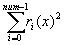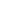SciMath Optimization

mgenlq   Nonlinear least squares

Description:

mgenlq finds a parameter vector x* of size n that minimizes the sum of squares function f(x):

f(x) = 1/2where: ri (x) is a twice continuously differentiable function of x

mgenlq requires a user function to compute ri (x).Synopsis:

#include<sci.h>

int mgenlq ( int num, int n, double * x, int (*rfun) ( ), int maxf, double est )

Input:

num: Number of components in r(x) (i.e number of observations), num ³ 1

n: Number of elements of vector x (number of parameters)

x: Array of size n, as the initial parameter vector x*

rfun: User function representing r(x) (Declared external):

int (*rfun)( int *num, int * n, double * x, int * in, double * fx )

Input:

num: Number of components in r(x) (i.e number of observations) (a pointer)

n: Number of elements of vector x (number of parameters) (a pointer )

x: Array of size n, as the initial parameter vector x*

in: Number of times this function has been called (a pointer)

output

in: Set to zero only if x is out of bounds, otherwise do not change in

fx: Value of r(x) for this vector x

maxf: Maximum number of function evaluations allowed

est: Minimization criteria. Minimization criteria will be met only when:

|| f(x) - f(x*) || £ est £ ||f(x)|| or when:

|| x-x* || £ est ( || x || + || x* || ), where x* is the solution (Objective vector for minimization) and || x || denotes the Euclidean norm of vector x ( See introduction )

Output:

x: Array of size n, the solution vector x*

Errors:

In case of a non fatal error, external integer sci_err_code is set to the corresponding error code. Non fatal errors are denoted by a N following the error code. (See Error Handling)

 Error Code Error Description ERRS1 n < 1 ERRS2 maxf < 1 ERRS3 est £ 0.0 ERRS4   N Singular convergence ( too many free parameters in x ) ERRS5   N False convergence ( est may be to small ) ERRS6   N Number of calls to user function exceeds maxf ERRS7   N f(x) could not be evaluated at the initial x vector

Category: Optimization/Minimization/Maximization